# Direct sum of connections

(diff) ← Older revision | Latest revision (diff) | Newer revision → (diff)
Suppose$M$ is a differential manifold and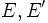$E,E'$ are vector bundles on$M$. Suppose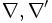$\nabla,\nabla'$ are connections on$E$ and$E'$ respectively. Then, we define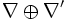$\nabla \oplus \nabla'$ as a connection on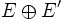$E \oplus E'$ given by: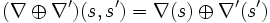$(\nabla \oplus \nabla')(s,s') = \nabla(s) \oplus \nabla'(s')$.